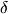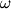# Towards lower bounds on locally testable codes via density arguments

###### Publication
Jun 12, 2011
[Work published prior to Yahoo]
###### Abstract

The main open problem in the area of locally testable codes (LTCs) is whether there exists an asymptotically good family of LTCs and to resolve this question it suffices to consider the case of query complexity 3. We argue that to refute the existence of such an asymptotically good family one should prove that the number of dual codewords of weight at most 3 is super-linear in the blocklength of the code.

The main technical contribution of this paper is an improvement of the combinatorial lemma of Goldreich et al.  which bounds the rate of 2-query locally decodable codes (LDCs) and is used in state-of-the-art rate-bounds for linear LDCs. The lemma of Goldreich et al. bounds the rate of 2-query LDCs of blocklength n in terms of the corruption parameter(n) --- this is the maximal number of corrupted codeword bits for which a (2-query) decoder can recover correctly every message bit (with high probability). Our combinatorial lemma gives nontrivial rate bounds for any corruption parameter(n)=(1), whereas the previous lemma works only for corruption parameter larger than logn. The study of LDCs with sublinear corruption parameter is also motivated by Dvir's  observation that sufficiently strong bounds on the rate of such LDCs imply explicit constructions of rigid matrices.